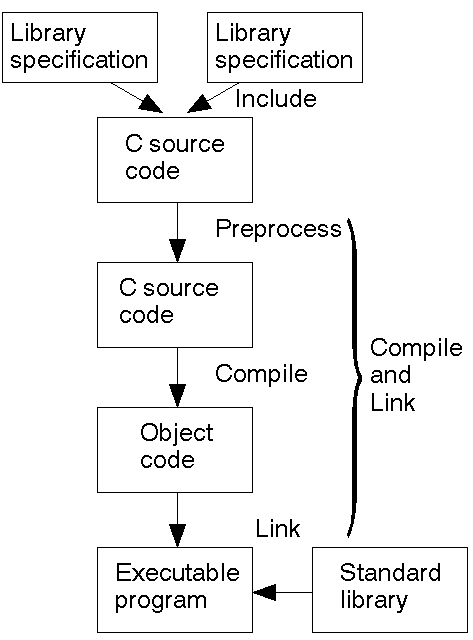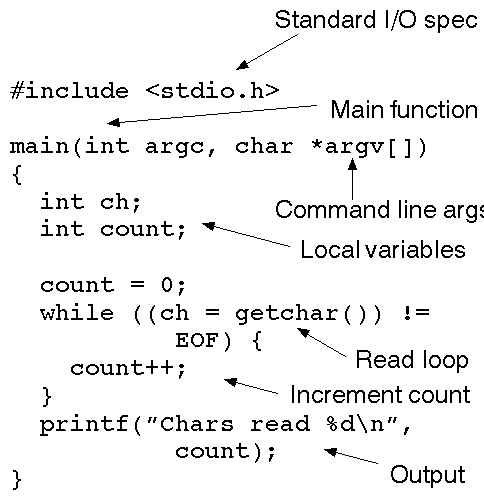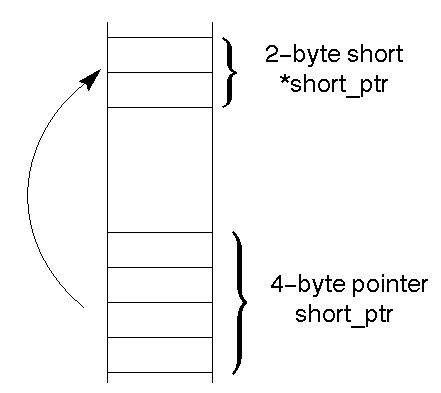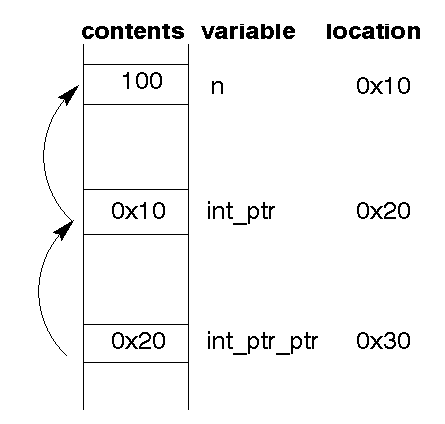# Introduction

This lecture looks at the overall structure of a C program, and then does a ``bottom up'' approach to the language. It looks at the basic data types and the common operations on them. It is important to note both the common features with other languages and the differences.

# The C language

The Language List (sometimes distributed on the InterNet) shows that over 5000 programming languages have been invented. Why look at this one?

The first version of Unix was written in PDP-11 Assembler. The C language was written to make it easier to manage Unix source code. It was designed as a language to write Operating Systems. IBM's OS/2 was originally written largely in Assembler, but is now mainly in C. Windows NT from Microsoft is mainly written in C. The language is very successful for writing Operating Systems.

So why would application programmers use it? The Application Programmers Interfaces (API) to Unix, OS/2 and Windows NT define a C language interface to the OS. If you want to open a file for reading, there is a C routine to do it. If you want to create a new process, there is a C routine to do it. So if you want to write a program that makes use of OS services, it is easiest to access these services through C.

There are often other language interfaces to the OS. For example, work is well advanced on an Ada interface to Unix. The main API for the Burroughs A9 is defined in Algol. The main API for the Burroughs B25s was in Pascal.

There are a large number of free and commercial libraries written in C, such as Microsoft Windows, the X Window system, maths packages, database routines, communications systems etc.

Despite its popularity, C has many unpleasant features. It is very easy to write programs that do not work (called ``shooting yourself in the foot''). Add comment

# Structure of C programs

A simple C program consists of one file. To create an executable from this, it goes through a number of steps, some involving other files.More complex programs may

• Use more source files
• Use other libraries.
Example: A program to count the number of characters read from standard input.

The components of this are:Add comment

# Scalar data types

## integers

Integers may be short, ordinary length (default) or long; signed (default) or unsigned int i, j, k; unsigned short l; The only constraint is that sizeof(short int) <= sizeof(int) <= sizeof(long int)

Ordinary decimal notation may be used, or octal (prefixed by 0), or hex (prefixed by 0x). i = 16; /* decimal */ j = 020; /* octal */ k = 0x10 /* hex */ Add comment

## Characters

Type char is a subset of int. It is unspecified as to what subset. However, ``` char + EOF ``` is a subset of ``` int ```
This often causes confusion about whether to declare variables as char or int.

Characters are generally enclosed in single quotes. Special characters such as newline have an ``escape'' representation

ch1 = 'A'; ch2 = 'a'; ch3 = '\n'; /* newline */ ch4 = '\t'; /* tab */ Add comment

## Pointers

Given any type (integer, char, structure, etc), you can have a pointer to that type. A pointer is the address of data. short int *short_ptr; char *char_ptr;The address of a variable is found by using the `&' operator. To dereference a pointer (find the value at the address) use the `*' operator

You do sometimes get indirect addressing int n; int *int_ptr; int **int_ptr_ptr; int_ptr = &n; int_ptr_ptr = &int_ptr; **int_ptr_ptr = 100; gives## Enumerated types

Define enumerated types by the `enum' keyword enum colour {red, green, amber}; and variables of these types by enum colour traffic_light; Use them as normal traffic_light = red; if (traffic_light == red) ...

## Synonyms for types

Create new names for types by `typedef' typedef long int * big_ptr_t; and then declare variables by big_ptr_t p; Add comment

## Boolean

There is no type Boolean. The integer 0 is Boolean False, and any other integer is True. (NB: Not the same way round as the shells.)

This infinite loop program prints lots of "true", but when the char wraps around to zero, prints "false":

## void

void has no type. It is used as a generic pointer type when you need one, or for functions that return no value.
e.g. sort an array of something - integers, chars, strings, etc, without specifying exactly what is being sorted. (This is done much better with Ada generic packages or in OO languages by virtual classes.) Add comment

# Expressions

Everything in C is an expression. x + y x = y The value of x+y is the sum. The value of x=y is x.

Expressions may be nested

x + y + z x = y = z You need to know the associativity rules for such expressions. Is 8 / 4 / 2 (8/4)/2 or 8/(4/2)?

Equality associates right to left:

(x = y = z) == (x = (y = z)) There are the usual arithmetic and relational operators. NB: the Boolean equality test is `==': if (x == y) ... Be suspicious of if (x = y) ... It is legal, and may be correct (the value of x=y is x, and if this is integral then it has a Boolean value), but is probably a semantic error.

The inequality operator is `!='.

A huge amount of code combines an assignment statement (typically through a function call) with a Boolean test:

if ((ch = getchar()) != EOF) ... Note the brackets to ensure the assignment is done before the inequality.

The logical operators are
` &&` - cand (Ada AND THEN)
` ||` - cor (Ada OR ELSE)
` != ` - not equal
The operators are ``short circuit'' ones, so the following executes correctly

## Special operators

There are special increment and decrement operators:
`n++`
value is n.
increment n by one after
`n--`
value is n.
decrement n by one after
`++n`
increment n by one before.
value is new n
`--n`
decrement n by one before.
value is new n
Typical slightly cryptic code is while (n--) ...

And new forms of assignment n += 1 /*n = n + 1*/ n -= 3 /*n = n - 3*/ The relations between different types of operators is very important *p++ == *(p++) means ``value of p++ is p, then increment (pointer) p by one. value of *p++ is value pointed to by p, then p moves to next address.'' (*p)++ is less common, but means ``value of what p is pointing to is incremented by one.'' Add comment

### Example

read in a list of characters until end of file, and sum all the digit characters.

# Summary

This lecture has looked at the overall structure of a C program. It examined the basic data types of C, and looked at common operations on them. Several things are common with other languages such as Ada, but there are differences of both a syntactic and semantic nature. You need to pay attention to these.

# Confusion about `int` or `char`

If you want to declare a variable that should hold chars, should it be of type `char` or `int`? The function `getchar()` returns chars, so why is it declared to return `int`?

If the value is `char+EOF` then it should be declared of type `int`, because that is a superset of `char+EOF`.

If the value can never be `EOF`, then it should be of type `char`.

The function `getchar()` can return EOF. So it must be of type `int`. If a variable is assigned the value from `getchar()` then it can take EOF, so it must be of type `int` also. However, if it is not EOF, then type `char` is ok:

int ich; if ((ich = getchar()) != EOF) { /* cant be EOF now */ char ch = (char) ich; ... } Add comment